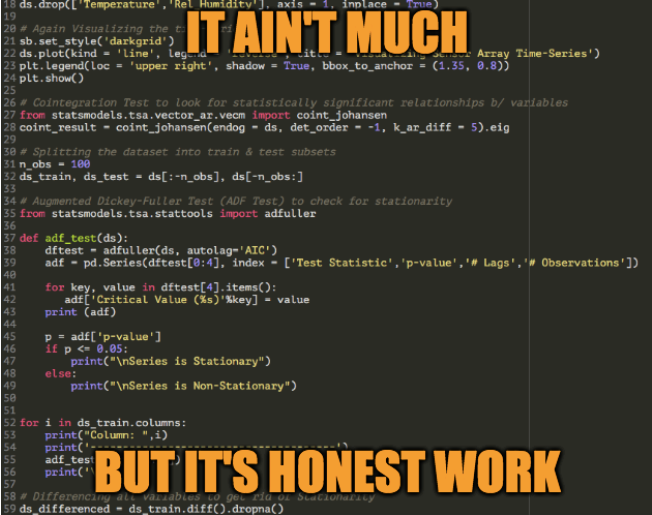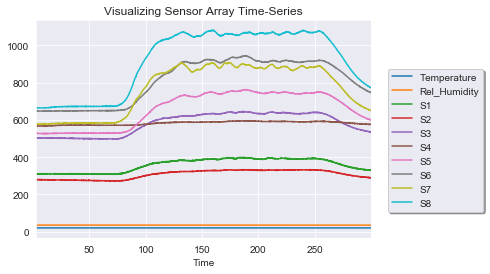1659699736

# Time Series Analysis Models and Techniques | Intellipaat

There are numerous methods for studying data, just as there are numerous types and models. The top three are listed below.

Want to learn about Data Analyst . So, look no further, Learn from Data Analytics Course

• Box-Jenkins ARIMA models: These univariate models are used to forecast future data points of variables and to gain a better understanding of a single time-dependent variable, such as temperature over time. The data must be stationary for these models to function. Analysts must take into consideration and eliminate as many variations and seasonality's from previous data points as they can. Thanks to the ARIMA model, moving averages, seasonal difference operators, and autoregressive terms can all be taken into account.
• Box-Jenkins Multivariate Models: Box-Jenkins Multivariate models are used to examine multiple time-dependent variables over time, such as temperature and humidity.
• Method of Holt-Winters: A method of exponential smoothing is the Holt-Winters approach. If seasonality is present in the data points, it is intended to predict outcomes.

## Buddha Community1623292080

## Getting started with Time Series using Pandas

### An introductory guide on getting started with the Time Series Analysis in Python

Time series analysis is the backbone for many companies since most businesses work by analyzing their past data to predict their future decisions. Analyzing such data can be tricky but Python, as a programming language, can help to deal with such data. Python has both inbuilt tools and external libraries, making the whole analysis process both seamless and easy. Python’s Panda s library is frequently used to import, manage, and analyze datasets in various formats. However, in this article, we’ll use it to analyze stock prices and perform some basic time-series operations.

#data-analysis #time-series-analysis #exploratory-data-analysis #stock-market-analysis #financial-analysis #getting started with time series using pandas1595685600

## What is Time Series Forecasting?

In this article, we will be discussing an algorithm that helps us analyze past trends and lets us focus on what is to unfold next so this algorithm is time series forecasting.

What is Time Series Analysis?

In this analysis, you have one variable -TIME. A time series is a set of observations taken at a specified time usually equal in intervals. It is used to predict future value based on previously observed data points.

Here some examples where time series is used.

2. Understand the past behavior
3. Plan future
4. Evaluate current accomplishments.

Components of time series :

1. Trend: Let’s understand by example, let’s say in a new construction area someone open hardware store now while construction is going on people will buy hardware. but after completing construction buyers of hardware will be reduced. So for some times selling goes high and then low its called uptrend and downtrend.
2. **Seasonality: **Every year chocolate sell goes high during the end of the year due to Christmas. This same pattern happens every year while in the trend that is not the case. Seasonality is repeating same pattern at same intervals.
3. Irregularity: It is also called noise. When something unusual happens that affects the regularity, for example, there is a natural disaster once in many years lets say it is flooded so people buying medicine more in that period. This what no one predicted and you don’t know how many numbers of sales going to happen.
4. Cyclic: It is basically repeating up and down movements so this means it can go more than one year so it doesn’t have fix pattern and it can happen any time and it is much harder to predict.

Stationarity of a time series:

A series is said to be “strictly stationary” if the marginal distribution of Y at time t[p(Yt)] is the same as at any other point in time. This implies that the mean, variance, and covariance of the series Yt are time-invariant.

However, a series said to be “weakly stationary” or “covariance stationary” if mean and variance are constant and covariance of two-point Cov(Y1, Y1+k)=Cov(Y2, Y2+k)=const, which depends only on lag k but do not depend on time explicitly.

#machine-learning #time-series-model #machine-learning-ai #time-series-forecasting #time-series-analysis1623226129

## Time Series Analysis Using ARIMA Model With Python

Time series_ is a sequence of time-based data points collected at specific intervals of a given phenomenon that undergoes changes over time. In other words, time series is a sequence taken at consecutive equally spaced points in the time period._

As a example, we can present few time series data sets in different domains such as pollution levels, Birth rates, heart rate monitoring, global temperatures and Consumer Price Index etc. At the processing level, above datasets are tracked, monitored, down sampled, and aggregated over time.

There are different kind of time series analysis techniques in the big data analytical field. Among them few are,

• Autoregression (AR)
• Moving Average (MA)
• Autoregressive Moving Average (ARMA)
• Autoregressive Integrated Moving Average (ARIMA)
• Seasonal Autoregressive Integrated Moving-Average (SARIMA)

ARIMA Model

ARIMA Model is simple and flexible enough to capture relationship we would see in the data and It aims to explain the autocorrelation between the data points using past data. We can decompose the ARIMA model as follow to grab the key elements of it.

• **AR: _Auto regression. _**This is a model that uses the dependent relationship between the data and the lagged data.
• **I:_ Integrated. _**The use of differencing of raw observations (e.g. subtracting an observation from an observation at the previous time step) in order to make the time series stationary.
• **MA: _Moving average. _**A model that uses the relationship between the observations and the residual error from the moving average model applied to lagged observations.

Dataset Explanation

Exploratory Analysis

#python #time-series-analysis #pandas #forecasting #arima #time series analysis using arima model with python1601550000

## How to model a time series through a SARIMA model

In this tutorial I will show you how to model a seasonal time series through a SARIMA model.

Here you can download the Jupyter notebook of the code described in this tutorial.

## Getting Started

### Convert the dataset into a time series

In this example we will use the number of tourist arrivals to Italy. Data are extracted from the European Statistics: Annual Data on Tourism Industries. Firstly, we import the dataset related to foreign tourists arrivals in Italy from 2012 to 2019 October and then we convert it into a time series.

In order to perform the conversion to time series, two steps are needed:

• the column containing dates must be converted to datetime. This can be done through the function `to_datetime()`, which converts a string into a datetime.
• set the index of the dataframe to the column containing dates. This can be done through the function `set_index()` applied to the dataframe.

#data-analysis #data-science #sarima #time-series-analysis #time-series-forecasting1594073100

## Simple Multivariate Time-Series Forecasting

This tutorial was supposed to be published last week. Except I couldn’t get a working (and decent) model ready in time to write an article about it. In fact, I’ve had to spend 2 days on the code to wrangle some semblance of useful and legible output from it.

But I’m not mad at it (now). This is the aim of my challenge here and truthfully I was getting rather tired of solving all the previous classification tasks in a row. And the good news is I’ve learned how to model the data in a suitable format for processing, conducting exploratory data analysis on time-series data and building a good (the best I could come up with, like, after 2 days) model.

So I’ve also made a meme to commemorate my journey. I promise the tutorial is right on the other side of it.Yes, I made a meme of my own code.

_About the Dataset: __The Gas Sensor Array Dataset, download from here**, _**consists of 8 sensor readings all set to detect concentration levels of a mixture of Ethylene gas with either Methane or Carbon Monoxide. The concentration levels are constantly changing with time and the sensors record this information.

Regression is one other possible type of solution that can be implemented for this dataset, but I deliberately chose to build a multivariate time-series model to familiarize myself with time-series forecasting problems and also to set more of a challenge to myself.

Time-Series data continuosuly varies with time. There may be one variable that does so (univariate), or multiple variables that vary with time (multivariate) in a given dataset.

Here, there are 11 feature variables in total; 8 sensor readings (time-dependent), Temperature, Relative Humidity and the Time (stamp) at which the recordings were observed.

As with most datasets in the UCI Machine Learning Repository, you will have to spend time cleaning up the flat files, converting them to a CSV format and insert the column headers at the top.

T

his is going to be a long tutorial with explanations liberally littered here and there, in order to explain concepts that most beginners might not be knowing. So in advance, thank you for your patience and I’ll keep the explanations to the point and as short as possible.

# 1) Exploratory Data Analysis -

Before heading into the data preprocessing part, it is important to visualize what variables are changing with time and how they are changing (trends) with time. Here’s how.Time Series Data Plot

``````# Gas Sensing Array Forecast with VAR model

# Importing libraries
import numpy as np, pandas as pd
import matplotlib.pyplot as plt, seaborn as sb

# Importing Dataset
ds = df.drop(['Time'], axis = 1)

# Visualize the trends in data
sb.set_style('darkgrid')
ds.plot(kind = 'line', legend = 'reverse', title = 'Visualizing Sensor Array Time-Series')
plt.legend(loc = 'upper right', shadow = True, bbox_to_anchor = (1.35, 0.8))
plt.show()

# Dropping Temperature & Relative Humidity as they do not change with Time
ds.drop(['Temperature','Rel_Humidity'], axis = 1, inplace = True)

# Again Visualizing the time-series data
sb.set_style('darkgrid')
ds.plot(kind = 'line', legend = 'reverse', title = 'Visualizing Sensor Array Time-Series')
plt.legend(loc = 'upper right', shadow = True, bbox_to_anchor = (1.35, 0.8))
plt.show()
view raw
gsr_data_prepocessing.py hosted with ❤ by GitHub
``````

It is evident that the ‘Temperature’ and ‘Relative Humidity’ variables do not really change with time at all. Therefore I have dropped the columns; time, temperature and rel_humidity from the dataset, to ensure that it only contains pure, time-series data.

# 2) Checking for Stationarity:

Non-stationary data has trends that are present in the data. We will have to eliminate this property because the Vector Autoregression (VAR) model, requires the data to be stationary.

A Stationary series is one whose mean and variance do not change with time.

One of the ways to check for stationarity is the ADF test. The ADF test has to be implemented for all the 8 sensor readings column. We’ll also split the data into train & test subsets.

#multivariate-analysis #time-series-forecasting #data-science #machine-learning #time-series-analysis #data analysis